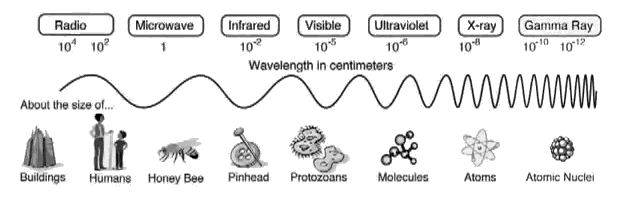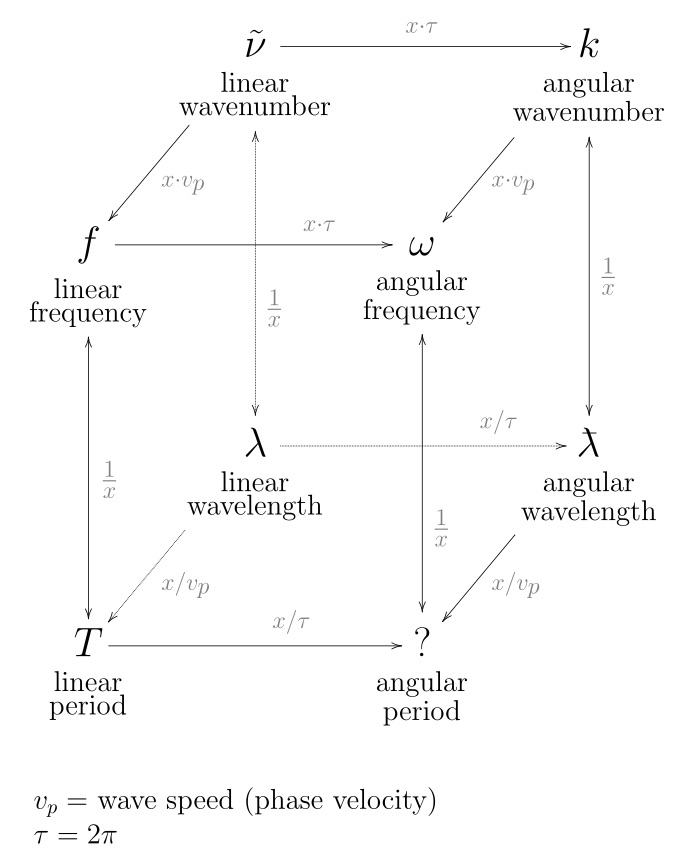# WaveLengthRelative Wavelengths

In physics, the wavelength is the spatial period of a periodic wave — the distance over which the wave's shape repeats. It is thus the inverse of the spatial frequency. Wavelength is usually determined by considering the distance between consecutive corresponding points of the same phase, such as crests, troughs, or zero crossings and is a characteristic of both traveling waves and standing waves, as well as other spatial wave patterns. Wavelength is commonly designated by the Greek letter lambda (λ). The term wavelength is also sometimes applied to modulated waves, and to the sinusoidal envelopes of modulated waves or waves formed by interference of several sinusoids.

Assuming a sinusoidal wave moving at a fixed wave speed, wavelength is inversely proportional to frequency of the wave: waves with higher frequencies have shorter wavelengths, and lower frequencies have longer wavelengths.

Wavelength depends on the medium (for example, vacuum, air, or water) that a wave travels through.

Examples of wave-like phenomena are sound waves, light, water waves and periodic electrical signals in a conductor. A sound wave is a variation in air pressure, while in light and other electromagnetic radiation the strength of the electric and the magnetic field vary. Water waves are variations in the height of a body of water. In a crystal lattice vibration, atomic positions vary.

Wavelength is a measure of the distance between repetitions of a shape feature such as peaks, valleys, or zero-crossings, not a measure of how far any given particle moves. For example, in sinusoidal waves over deep water a particle near the water's surface moves in a circle of the same diameter as the wave height, unrelated to wavelength. The range of wavelengths or frequencies for wave phenomena is called a spectrum. The name originated with the visible light spectrum but now can be applied to the entire electromagnetic spectrum as well as to a sound spectrum or vibration spectrum. Wikipedia, WavelengthDistance measured along line of propagation, between two points that are in phase on adjacent waves.
[Anderson, Philip C.; The Spectral Energy Value System; Applied Spectroscopy, Vol. 29, #1, 1975.]

To ascertain the wavelength of any given sound - divide the velocity of sound by its vibration number.

The wavelength of any given sound, increases with the temperature.

The temperature remaining constant, the length of the sound wave determines the pitch of the sound produced. [Harris, T. F.; Hand Book of Acoustics, 5th edition; J. Curwen & Sons, London, 1903?]

The range of musical pitch is from about 40 to 4,000 vibrations per second

The length in space of one complete cycle of sound wave.

A = speed of sound/frequency = c/f

where A = wavelength in ft or m.

Wavelength can be calculated from the following equation:

Wavelength (meters) = Velocity 300,000,000 (meters/sec) / Frequency (cycles/sec)

If we substitute 1.5 MHz for the frequency as shown, then

200 meters = 300,000,000 / 1,500,000
[Hirschorn, Martin; Compendium of Noise Engineering - Part I Sound & Vibration Magazine, July 1987.; Sound & Vibration Magazine, July 1987.]

Hughes
On colours developing by the same laws as musical harmonies
—The physical properties of light and darkness briefly considered
—If the laws are correctly gained, harmonics of tones and of colours will agree
—Quotation from a lecture by Professor W. F. Barrett on the order of sonorous and luminous wave-lengths
—Fountain of musical harmonics, E root of B; in colours yellow and ultra-violet, being tints and shades of white and black
—All harmonics of sound and colour condense into a primo springing from the fountain
Multequivalency of tones and colours
Wünsch's views nearly one hundred years ago